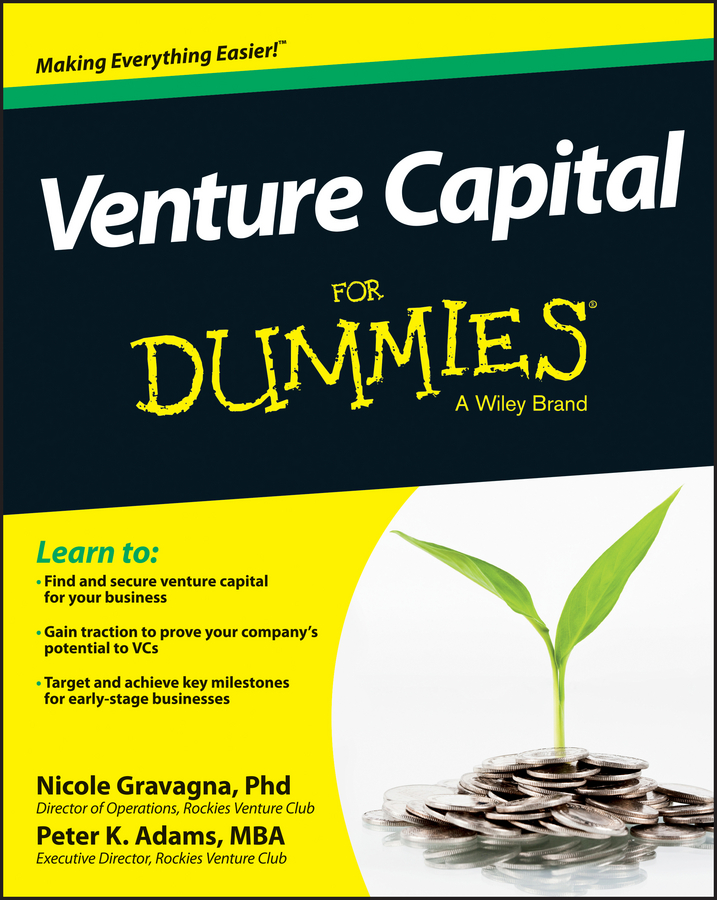##### Venture Capital For DummiesFew businesses are able to make major purchases without taking out loans. Businesses must pay interest, a percentage of the amount loaned, to whoever loans them the money, whether loans are for vehicles, buildings, or other business needs.

Some businesses loan their own money and receive interest payments as income. In fact, a savings account can be considered a type of loan because by placing your money in the account, you’re giving the bank the opportunity to loan that money to others. So the bank pays you for the use of your money by paying interest, which is a type of income for your company.

The financial institution that has your money will likely combine your money with that of other depositors and loan it out to other people to make more interest than it’s paying you. That’s why when the interest rates you have to pay on loans are low, the interest rates you can earn on savings are even lower.

Banks actually use two types of interest calculations:

• Simple interest is calculated only on the principal amount of the loan.

• Compound interest is calculated on the principal and on interest earned.

## Simple interest

Simple interest is simple to calculate. Here’s the formula for calculating simple interest:

Principal × interest rate × n = interest

To show you how interest is calculated, assume that someone deposited \$10,000 in the bank in a money market account earning 3 percent (0.03) interest for 3 years. So, the interest earned over 3 years is \$10,000 × .03 × 3 = \$900.

## Compound interest

Compound interest is computed on both the principal and any interest earned. You must calculate the interest each year and add it to the balance before you can calculate the next year’s interest payment, which will be based on both the principal and interest earned.

Here’s how you would calculate compound interest:

Principal × interest rate = interest for Year One
(Principal + interest earned) × interest rate = interest for Year Two
(Principal + interest earned) × interest rate = interest for Year Three

You repeat this calculation for all years of the deposit or loan. The one exception could be with a loan. If you pay the total interest due each month or year (depending on when your payments are due), there would be no interest to compound.

When working with large sums or high interest rates for long periods of time, compound interest can make a big difference in how much you earn or how much you pay on a loan.

Ideally, you want to find a savings account, certificate deposit, or other savings instrument that earns compound interest. But, if you want to borrow money, look for a simple interest loan.

Not all accounts that earn compound interest are created equally. Watch carefully to see how frequently the interest is compounded. If you can find an account where interest is compounded monthly, the interest you earn will be even higher.

Monthly compounding means that interest earned will be calculated each month and added to the principle each month before calculating the next month’s interest, which results in a lot more interest than a bank that compounds interest just once a year.